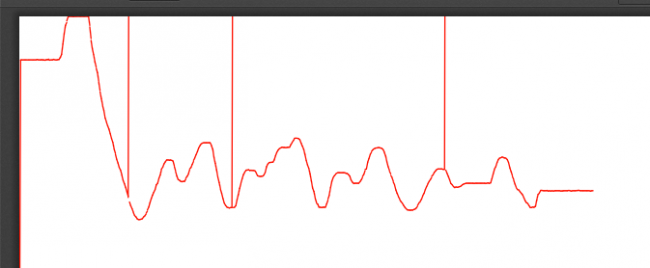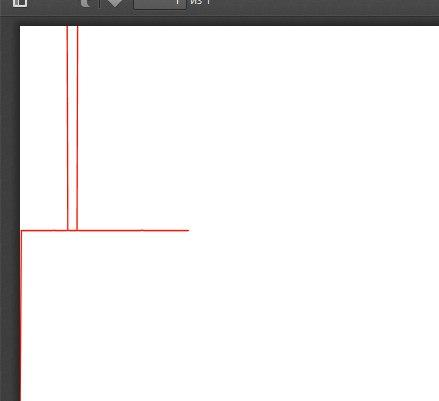#### Howdy, Stranger!

We are about to switch to a new forum software. Until then we have removed the registration on this forum.

# Unknown issue with drawing graph with data send by arduino to Raspberry Pi

edited October 2017

Hello!

I'm using arduino as ADC, which sends data through UART to Raspberry Pi model B, which draws a graph on 7'display, connected via HDMI.

So I've got a strange problem.Randomly straight lines appear from current measuring point to top of the screen.

I was thinking about mistakes or zero values or Nan values of something like that. But it is not-)

Lines drawn like that:

`line(xPos-1, height-lastfValue, xPos, height - fValue);`

I was trying to filter it out by using such a construction:

``````if(fValue <= 0 || (height - fValue) > height || ((fValue - lastfValue)*(fValue - lastfValue)/2) > lastfValue + 5 ){
fValue = lastfValue;
}
``````

It helped a little, but did't became a solution for my problem. So I tried to figure out a problem and added pdf export of what I see on the screen and logging values of "fValue" to console and find out:

The values:

``````145.7478
145.7478
145.7478
145.7478
145.7478
145.7478
145.7478
145.7478
145.7478
145.7478
145.7478
145.7478
145.7478
145.7478
145.7478
145.7478
145.7478
145.7478
145.7478
145.7478
145.7478
145.7478
145.7478
145.7478
145.7478
145.7478
145.7478
145.7478
145.7478
145.7478
145.7478
145.7478
145.7478
145.7478
145.7478
145.7478
145.7478
145.7478
145.7478
145.7478
145.7478
145.7478
145.7478
145.7478
145.7478
145.7478
145.7478
145.7478
145.7478
145.7478
145.7478
145.7478
145.7478
145.7478
145.7478
145.7478
145.7478
145.7478
145.7478
145.7478
145.7478
145.7478
145.7478
145.7478
145.7478
145.7478
145.7478
145.7478
145.7478
145.7478
145.7478
145.7478
145.7478
145.7478
145.7478
145.7478
145.7478
145.7478
145.7478
145.7478
145.7478
145.7478
145.7478
145.7478
145.7478
145.7478
145.7478
145.7478
146.04106
145.7478
145.7478
145.7478
145.7478
145.7478
145.7478
145.7478
145.7478
145.7478
145.7478
145.7478
145.7478
145.7478
145.7478
145.7478
145.7478
145.7478
145.7478
145.7478
145.7478
145.7478
145.7478
145.7478
145.7478
145.7478
145.7478
145.7478
145.7478
145.7478
145.7478
145.7478
145.7478
145.7478
``````

and the graph:As you can see there only one different value and two peaks on the graph.

Does anyone know how to deal with it and get a correct graph?

This code works the same way on my notebook..

Here is the code I use for processing on raspberry Pi:

``````import processing.serial.*;
import processing.pdf.*;

Serial myPort;        // The serial port
int xPos = 1;         // horizontal position of the graph

float fValue;
float lastfValue;
boolean newVal = false;

void setup () {
size(1000, 300);
// fullScreen();

println(Serial.list());
myPort = new Serial(this, Serial.list(), 115200);
myPort.bufferUntil('\n');
background(28,28,28);
beginRecord(PDF, "rawData.pdf");
}

void draw () {
//  if (newVal) {
stroke(255, 16, 0);
// size(100, 100, P3D);
// noSmooth();
//ellipse(xPos, height - fValue, 1, 1);
line(xPos-1, height-lastfValue, xPos, height - fValue);
println(fValue);
lastfValue = fValue;
//fValue = (fValue - 3);
//stroke(28, 28, 28);
//point(xPos, height - fValue);
if (++xPos >= width) {
xPos = 0;
background(28,28,28);
//    }
//    newVal = false;
}
}

void serialEvent (Serial myPort) {
String inString = myPort.readStringUntil('\n');
if (inString != null) {
inString = trim(inString);
fValue = float(inString);
fValue = map(fValue, 0, 1023, 0, height);
//if(fValue <= 0 || (height - fValue) > height || ((fValue - lastfValue)*(fValue - lastfValue)/2) > lastfValue + 5 ){
//fValue = lastfValue;
//}
}
//  newVal = true;
}
void keyPressed() {
if (key == ' ') {
endRecord();
exit();
}
}
``````

Here is the code for arduino Uno that I use as ADC:

``````// the setup routine runs once when you press reset:
void setup() {
// initialize serial communication at 9600 bits per second:
Serial.begin(9600);
}

// the loop routine runs over and over again forever:
void loop() {
// read the input on analog pin 0:
int sensorValue = analogRead(A0);
// print out the value you read:
Serial.println(sensorValue);
delay(1);        // delay in between reads for stability
}
``````
Tagged:

## Answers

• edit post, highlight code, press ctrl-o to format.

(refresh page, repeat until it looks right)

• Like that?-)

• code formatting also works for data...

• edited October 2017

Sorry about that I'm for first time here-)

• edited October 2017

I think it's something in here:

`````` void serialEvent (Serial myPort) {
String inString = myPort.readStringUntil('\n');
if (inString != null) {
inString = trim(inString);
fValue = float(inString);
fValue = map(fValue, 0, 1023, 0, height);
//if(fValue <= 0 || (height - fValue) > height || ((fValue - lastfValue)*(fValue - lastfValue)/2) > lastfValue + 5 ){
//fValue = lastfValue;
//}
}
//  newVal = true;
}
``````

Maybe during datatype conversion "map" function receives a value that is not a number.. Hm.. But how to filter it out?

• It's something about NaN on "map" function. I'll try to find out how to check for NaN..

• The delay in the Arduino loop is 1 mS. I don't know how long the analogRead takes, let's guess 1 mS. So every 2mS it's trying to transmit about 10 chars, each takes 1mS at 9600 so 10 mS total. There is some buffering in the serial system. Don't know what happens when the buffer fills, which it will. Very likely it just drops a few characters and messes up some numbers.

Better to set the Arduino repeat to sensible period related to what you are trying to measure. If this is shorter than 20 mS then you will have to use > 9600 baud. Then your plot will cross the screen in a very short time.

In Processing your x is 1 per incoming value, which is irregular at the moment, and late because of buffering. If there are no delays or gaps you can use the Arduino for timing like this, but IMO better to use time as your x distance.

Sign In or Register to comment.